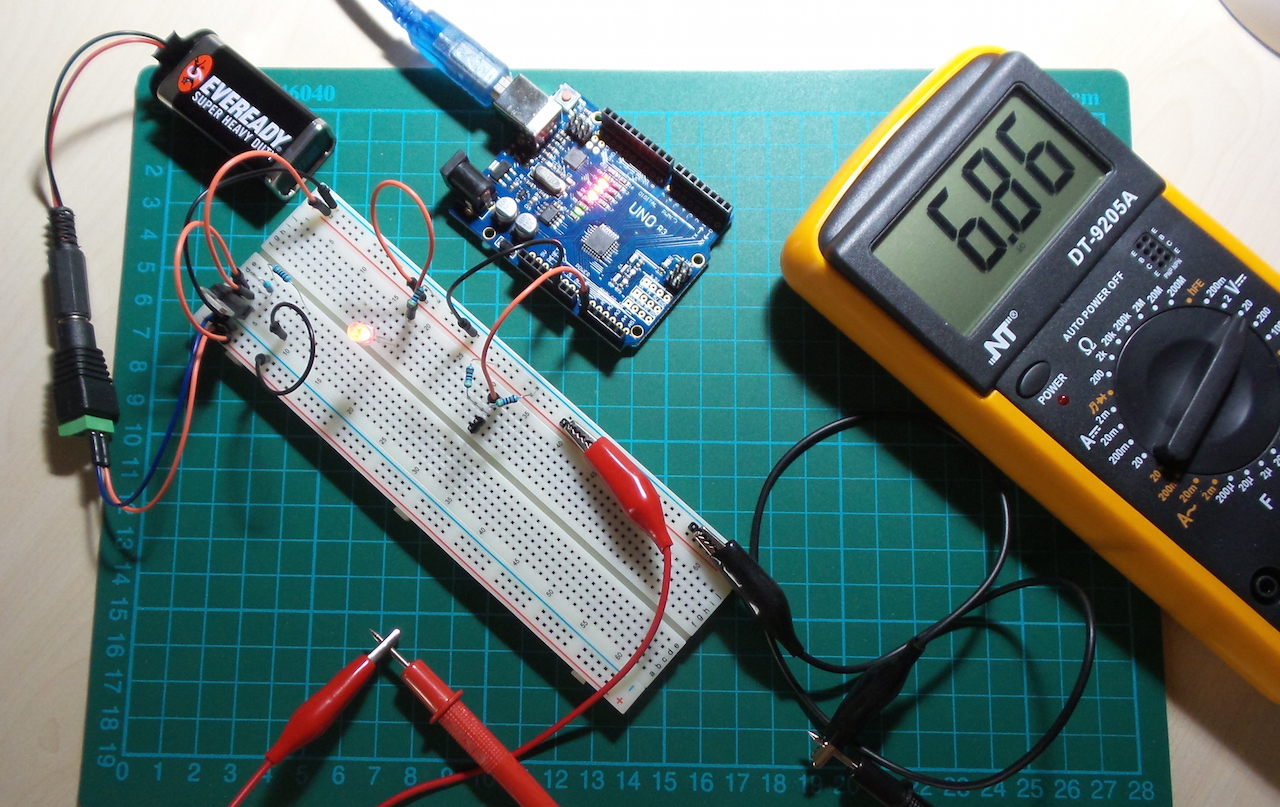### Project Notes

Demonstrates the LM317 voltage regulator, using an Arduino to measure the voltage supply and plot the results with Processing.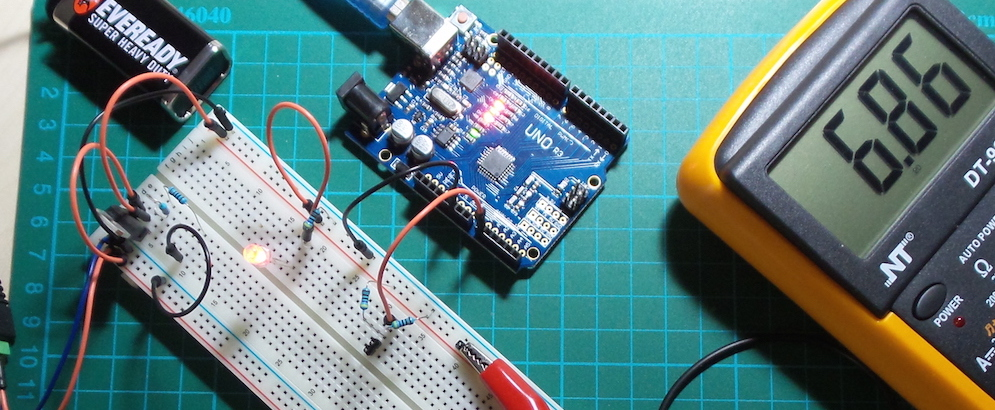The LM317 can be used to provide an adjustable voltage or current supply.

The circuit described here is a adjustable voltage supply. When used in this mode, the voltage depends on the values of R1 and R2:

``````Vout = 1.25 * ( 1 + R2/R1 )
``````

Various calculators are available on the web to figure out the various combinations, and I made my own

Check the datasheet to verify the formula for your specific chip. In a circuit, R2 could be fixed to provide a fixed voltage, or it can be a variable resistor to allow manipulation of the voltage. R2 could also be replaced by a sensor to convert sensor resistance to voltage.

### Baseline Test

Using known resistances, the measured value Vout is pretty close to spec for the chip I am using:

R1 R2 Vout Derivation
220R 1k 6.93 theoretical
222R 998R 6.87 theoretical, with actual resistances as measure with DMM
222R 998R 6.86 actual (DMM)
222R 998R 6.85 actual (Arduino)

### Using a Variable Resistor for R2

The circuit described in the build replaces the 1k R2 used in the reference circuit with a smaller 220R R2 and 50k R3 potentiometer, which allows a Vout ranging from 2.49V to the maximum supply possible (about 1.25V less than the input supply). Note that 50k is really too much for this circuit - but it is what I had on hand. Try it with a 10k pot for more precise control.

Here’s a sample trace with the pot being adjusted: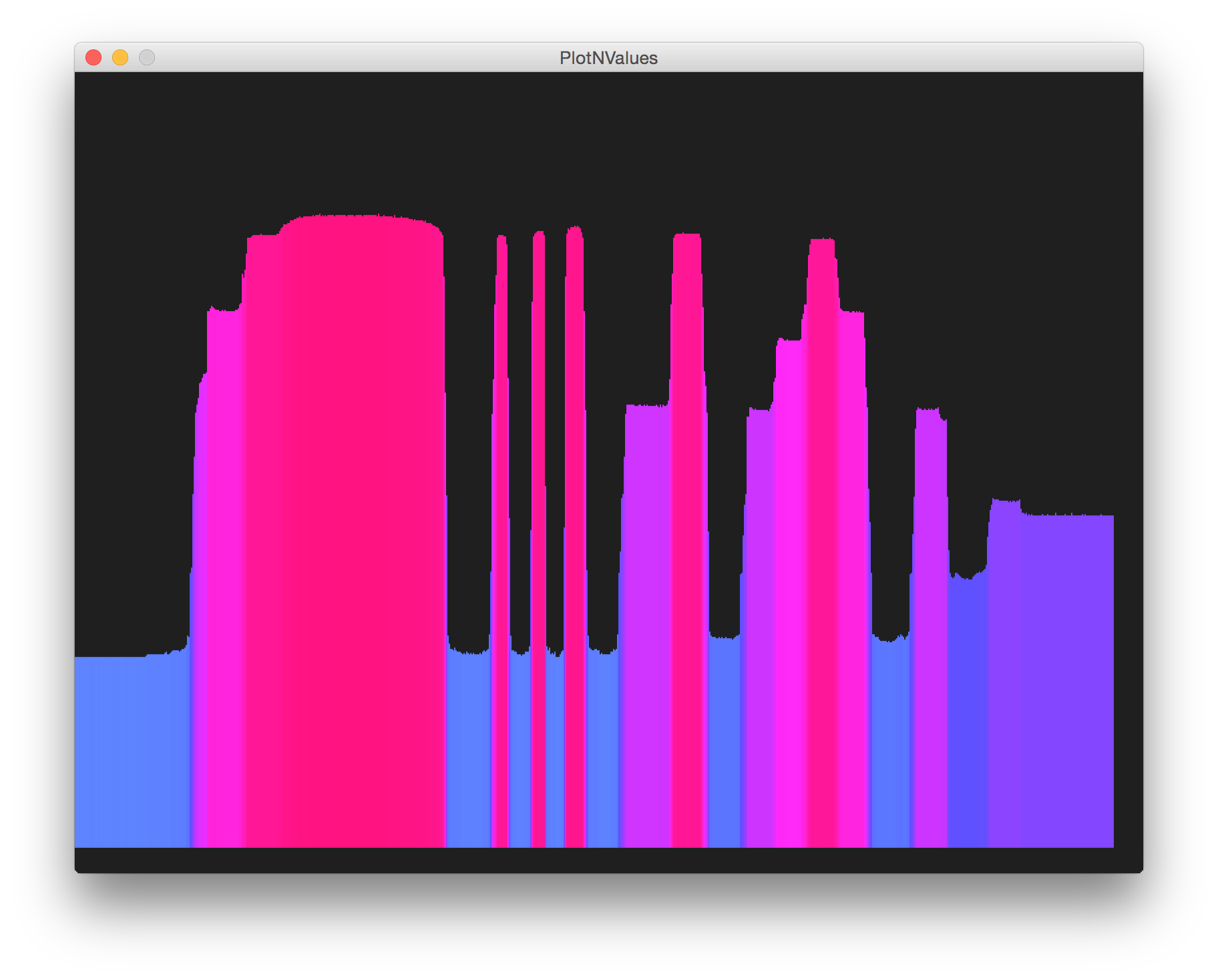### Measure Voltage with an Arduino

Reading voltages with analog pins presents two challenges:

• The internal ADC has 10 bit resolution (0 - 1023) with 2 bit precision, so the accuracy is 0.25%
• The internal reference voltage (i.e. what 1023 will equal in volts) is nominally 5V but in practice is not very accurate (the board used for this test runs at about 4.93V)

For our purposes here, the ADC accuracy is not a big problem. To overcome the reference voltage accuracy issue, the sketch scales the voltage reading according to the actual reference voltage of the board. This must be measured with a multimeter and the `vref_mv` constant changed to this value (in mV).

For more detail, John Errington’s Experiments with an Arduino includes an excellent study of Precise voltage measurement with the Arduino.

### Construction

The Arduino only acts as a measurement device in this circuit. Analog pin is used to read Vout via a voltage divider (Rd1/Rd2). The voltage divider is to ensure that the Arduino never sees more than half the battery voltage (4.5V) on the analog pin.

PlotNValues (a simple Processing sketch) reads the data from the serial port and plots the input and output value over time, with some coloration effects thrown in for good measure. In other words, we’re using Arduino and Processing as a basic oscilloscope! And it kind of works, mainly because the frequency is so low.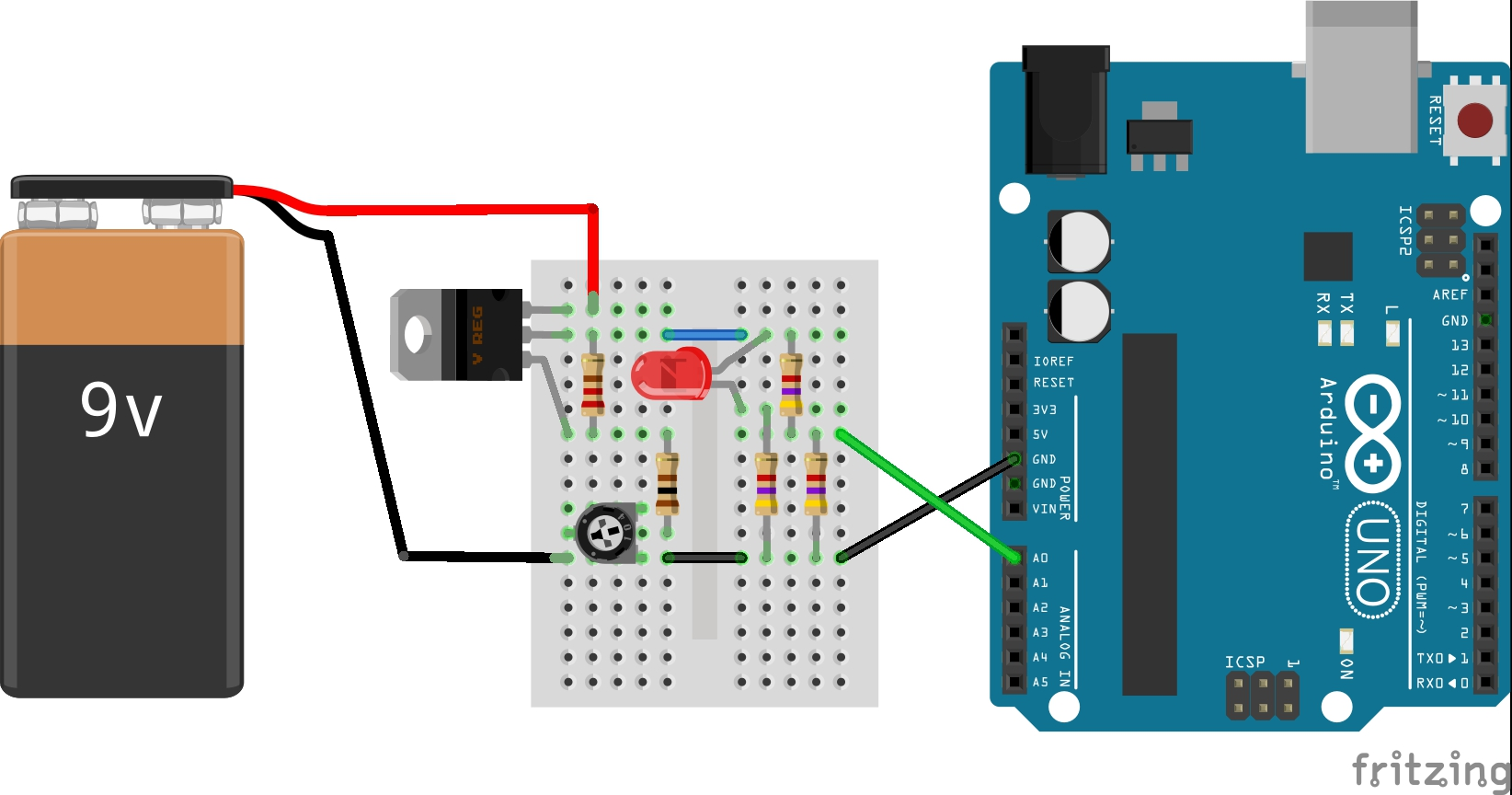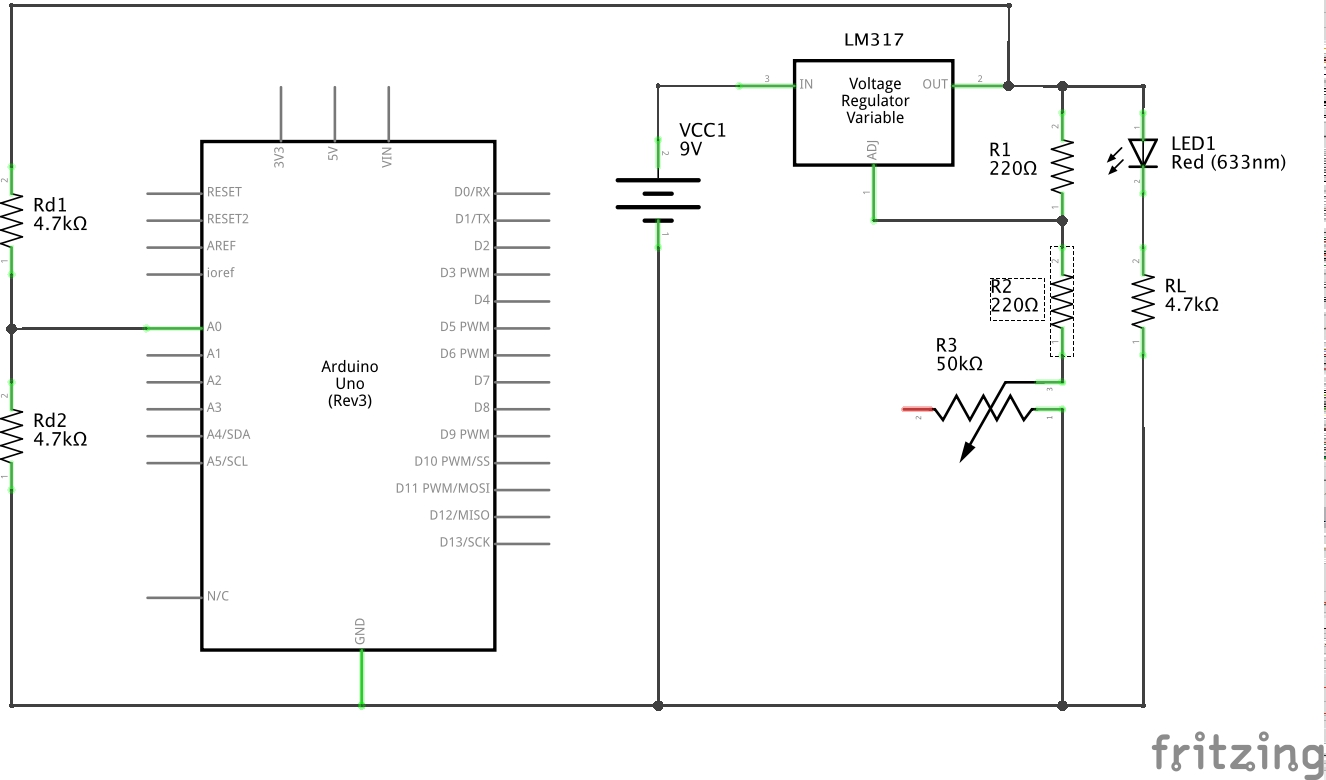Here’s the breadboard with the variable R2, note a little more spread out than my original Fritzing diagram: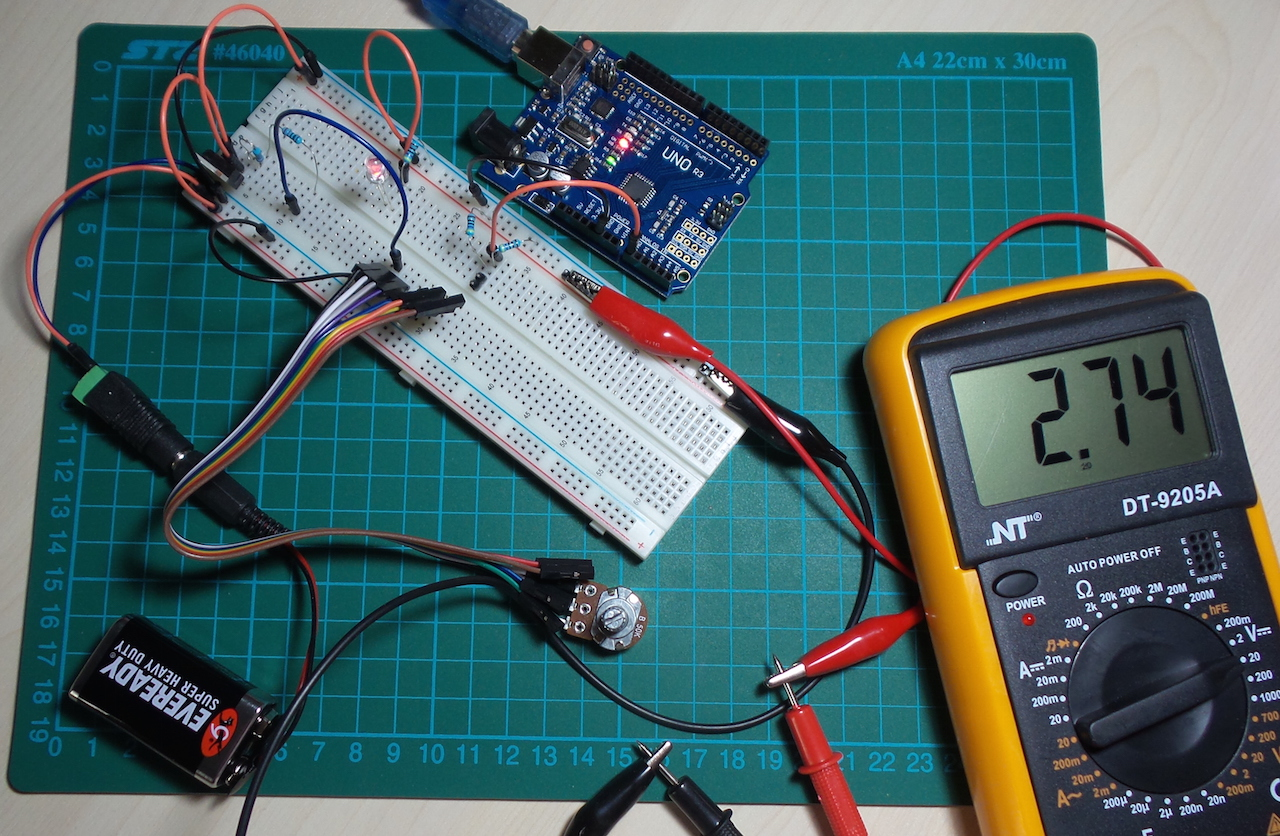Here’s the reference measurement with fixed/known R1 and R2 values: